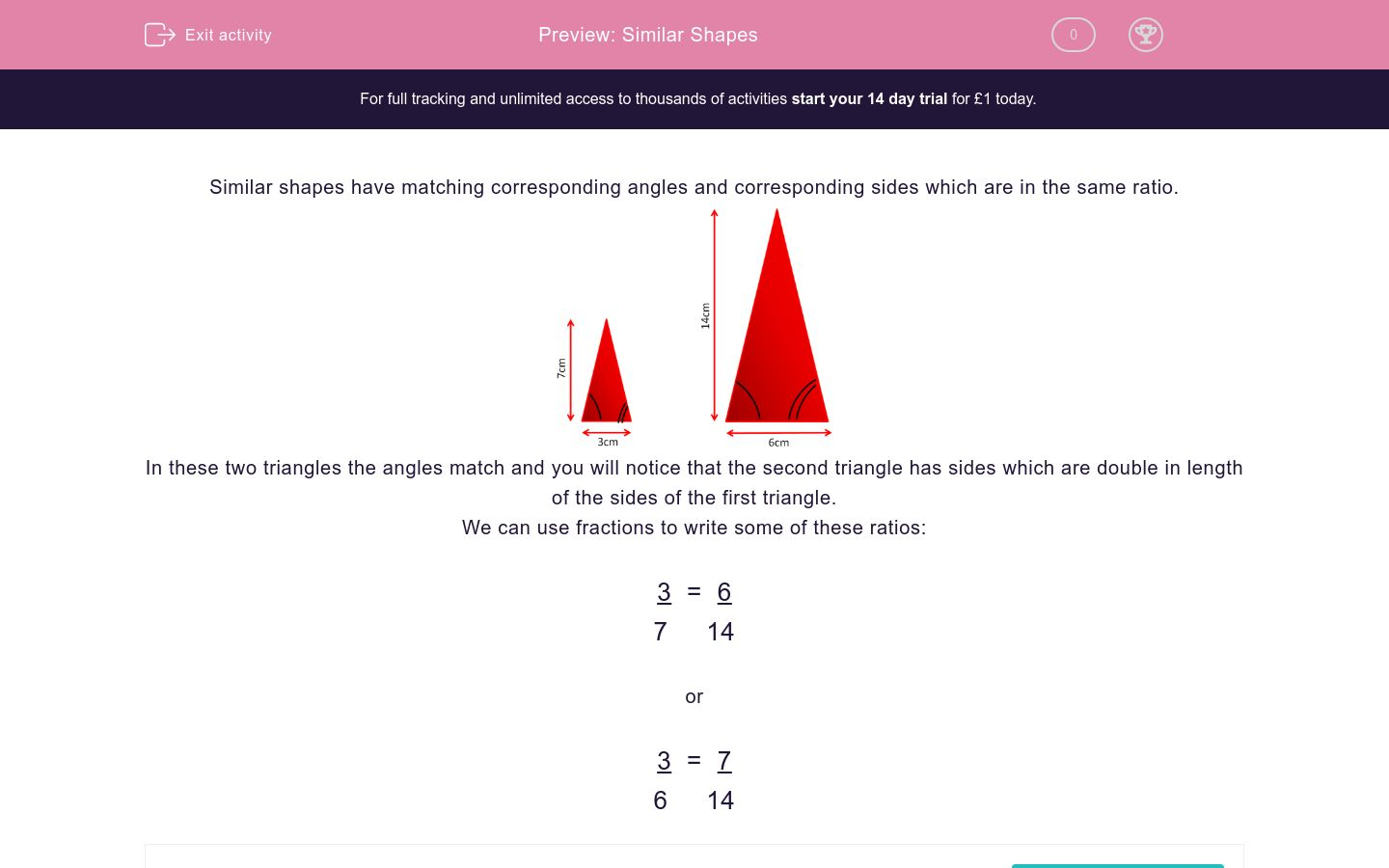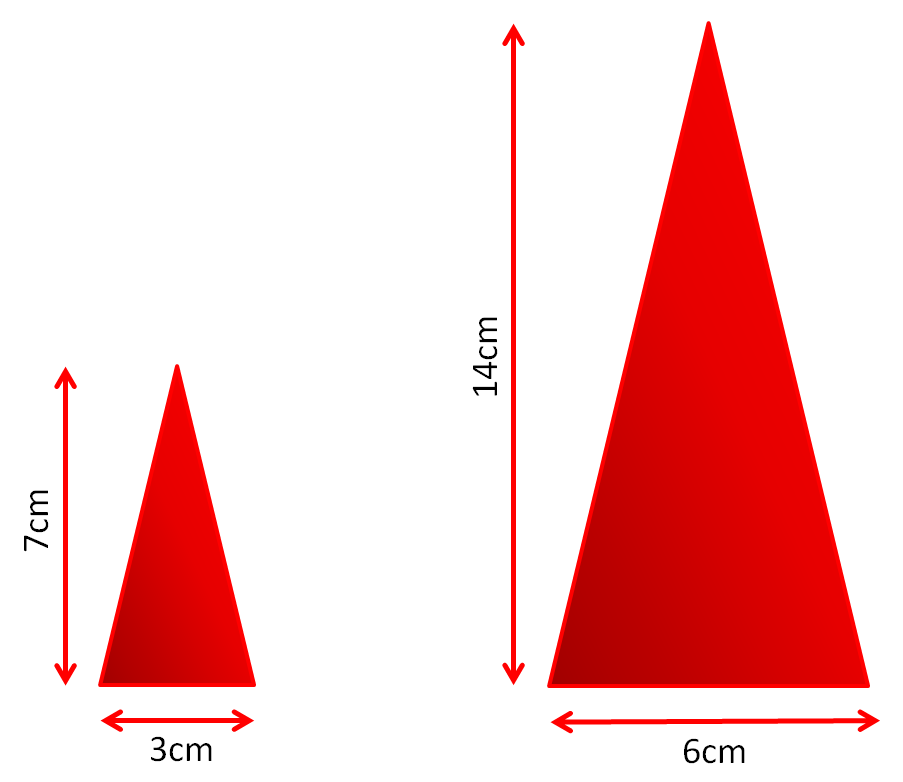# Similar Shapes

In this worksheet, students explore similar shapes and find the length of the missing sides.Key stage:  KS 2

Curriculum topic:   Maths and Numerical Reasoning

Curriculum subtopic:   2D Shapes: Triangles, Quadrilaterals and Polygons

Difficulty level:### QUESTION 1 of 10

Similar shapes have matching corresponding angles and corresponding sides which are in the same ratio.In these two triangles the angles match and you will notice that the second triangle has sides which are double in length of the sides of the first triangle.

We can use fractions to write some of these ratios:

3  =  6

7     14

or

3  =  7

6     14

State whether or not these shapes are similar.

The triangles' angles match.Yes

No

State whether or not these shapes are similar.

The triangles' angles match.Yes

No

State whether or not these rectangles are similar.Yes

No

State whether or not these rectangles are similar.Yes

No

State whether or not these rectangles are similar.Yes

No

These two shapes are similar.

State the missing length in cm.

(Just write the number)These two shapes are similar.

State the missing length in cm.

(Just write the number)These two shapes are similar.

State the missing length in cm.

(Just write the number)These two shapes are similar.

State the missing length in cm.

(Just write the number)These two shapes are similar.

State the missing length in cm.

(Just write the number)• Question 1

State whether or not these shapes are similar.

The triangles' angles match.No
• Question 2

State whether or not these shapes are similar.

The triangles' angles match.Yes
• Question 3

State whether or not these rectangles are similar.Yes
• Question 4

State whether or not these rectangles are similar.Yes
• Question 5

State whether or not these rectangles are similar.No
• Question 6

These two shapes are similar.

State the missing length in cm.

(Just write the number)10
• Question 7

These two shapes are similar.

State the missing length in cm.

(Just write the number)5
• Question 8

These two shapes are similar.

State the missing length in cm.

(Just write the number)2
• Question 9

These two shapes are similar.

State the missing length in cm.

(Just write the number)21
• Question 10

These two shapes are similar.

State the missing length in cm.

(Just write the number)3
---- OR ----

Sign up for a £1 trial so you can track and measure your child's progress on this activity.

### What is EdPlace?

We're your National Curriculum aligned online education content provider helping each child succeed in English, maths and science from year 1 to GCSE. With an EdPlace account you’ll be able to track and measure progress, helping each child achieve their best. We build confidence and attainment by personalising each child’s learning at a level that suits them.

Get started